#### You may also like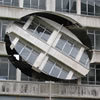### Turning the Place Over

As part of Liverpool08 European Capital of Culture there were a huge number of events and displays. One of the art installations was called "Turning the Place Over". Can you find our how it works?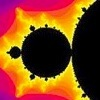### Where Art and Maths Combine

In this article, Rachel Melrose describes what happens when she mixed mathematics with art.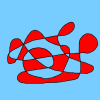### Colouring Curves Game

In this game, try not to colour two adjacent regions the same colour. Can you work out a strategy?

# Drawing Celtic Knots

##### Age 11 to 14 Challenge Level:

William from Barnton Community Primary School discovered that:

If there is a rectangular Celtic knot that is M by N then the number of ribbons is the highest common factor of M and N.

In this case M = 5 and N = 3 so the number of ribbons is 1.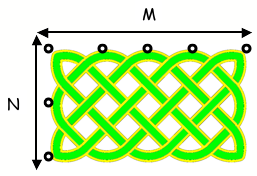Therefore, if a square Celtic knot has side length x, the number of different ribbons will be x.

In this case x = 4 so the number of ribbons is 4.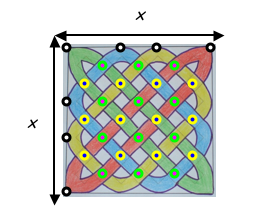The number of crossovers for a square Celtic knot is $$2x^2 - 2x$$ or $$2x (x - 1)$$

Students from Garden International School also worked on this problem. Here is what Kenn, Jong Woong, Jayme and Marana sent us.